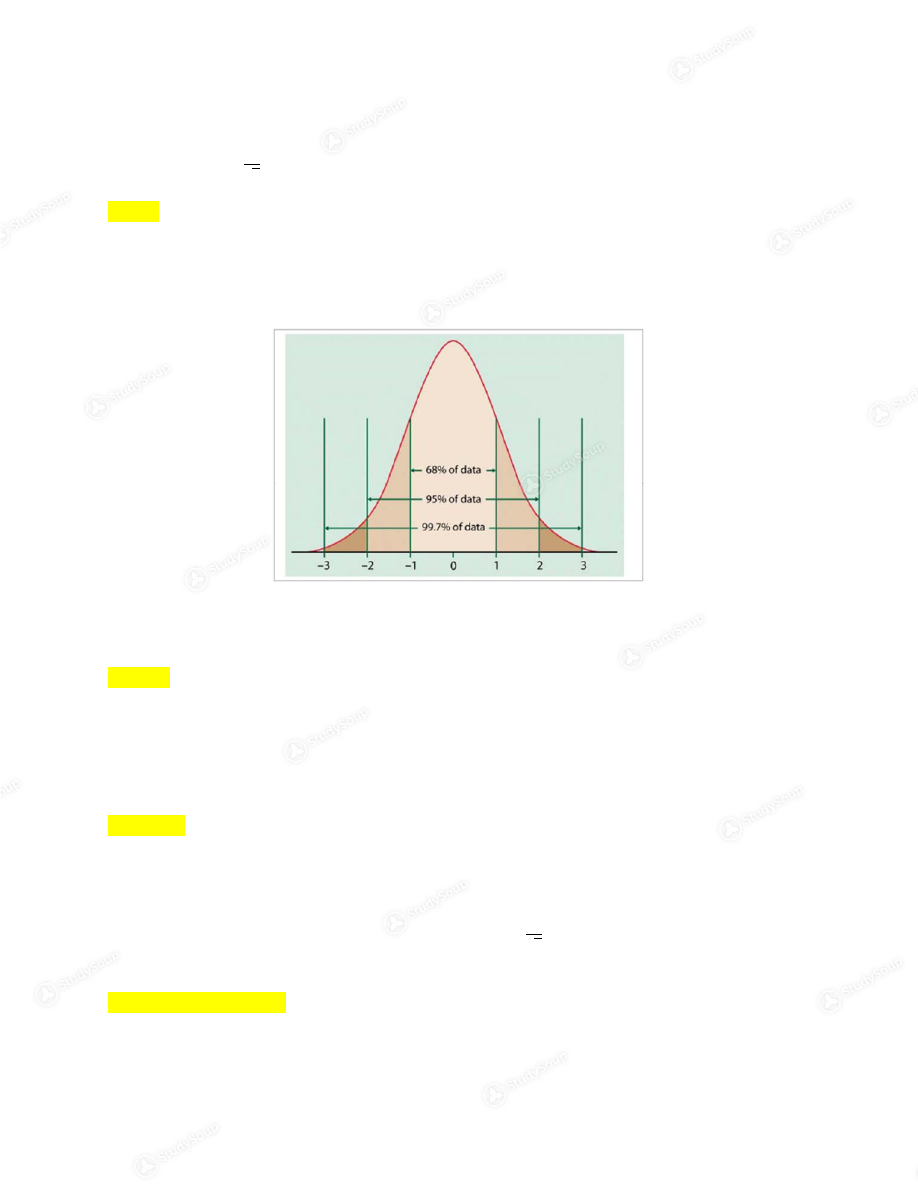Limited time offer 20% OFF StudySoup Subscription details

# FSU - POS 3713 - Exam three example test - Study Guide

### Created by: Leah Burkett Elite Notetaker

> > > > FSU - POS 3713 - Exam three example test - Study Guide

FSU - POS 3713 - Exam three example test - Study Guide

##### Description: includes example questions with answers and explanations
0 5 3 88 Reviews
This preview shows pages 1 - 3 of a 7 page document. to view the rest of the contentUnit exam #3 1. What is the key insight of the Central Limit Theorem? 2. Which of the following is not true about normal distributions? 3. Which of the following is not true about a sampling distribution? basis for statistical inference basis of statistical inference NOT common to
everyday examples. 68% of curve within 1 std dev.
away from mean.4. If a mean age of a sample of 100 randomly chosen individuals is 48 and the standard deviation of the sample is 16, what is the standard error of the mean?
Recall that
. The following 2 questions refer to this figure: 5. How large, in %, is the area to the left of (standard errors)? 6. How large, in %, is the area to the left of (standard errors)? 7. If, for a sample in which the subjects are randomly chosen, the mean income is \$45,000, the sample size is 1600, the standard deviation of the sample is \$4,000, and
the standard error of the mean is \$100, what, approximately, is the 95% confidence
interval for the population mean? Recall that
.   n=100
SD= 16
mean= 48  16/square root of 100 = 1.6
99.7 - 68 then divide by 2 = ~16% answer has to be between 95% and 99.7%
which is 97.5%
mean= 45,000
n= 1600
STD: 4000
SE: 100 Y +/- 2 x SE
Y+/- 2 x 100
200 + 45000
200 - 450008. If, for a sample in which the subjects are randomly chosen, the mean income is \$1,000, the sample size is 100, the standard deviation of the sample is \$100, and the
standard error of the mean is \$10, what, approximately, is the 99.7% confidence
interval for the population mean? Recall that
.   9. Suppose a reputable pollster reports that, on the basis of a random sample of
American adults, the President's approval rating is 50%, and that the margin of error
is plus or minus 4%. What is the correct interpretation of this reported result? 10. The process of statistical inference is defined as: 11. Which of the following is generally not an essential component of the process of
statistical inference?
12. Which type of sample is likely to be most useful for the process of statistical inference? mean= 1000
n= 100
STD= 100
SE= 10 Y+/- 3 x Se
y +/- 3 x 10
1000 + 30=
1030
1000- 30= 970
approval rating = 50%
ME= 4
50+4= 54
50-4= 46
the process of making
population characteristic based
on out knowledge of the sample
characteristic.
just need data for sample the most important
thing is that it is
randomly collected

This is the end of the preview. Please to view the rest of the contentJoin more than 18,000+ college students at Florida State University who use StudySoup to get ahead
7 Pages 72 Views 57 Unlocks
• Notes, Study Guides, Flashcards + More!Join more than 18,000+ college students at Florida State University who use StudySoup to get ahead
##### Description: includes example questions with answers and explanations
7 Pages 72 Views 57 Unlocks
• Notes, Study Guides, Flashcards + More!
×

I don't want to reset my password

Need help? Contact support

Need an Account? Is not associated with an account
We're here to help